Plotting polar curves in Python

• Last Updated : 22 Jun, 2020

A point in polar co-ordinates is represented as (r, theta). Here, r is its distance from the origin and theta is the angle at which r has to be measured from origin. Any mathematical function in the Cartesian coordinate system can also be plotted using the polar coordinates.

Modules required

• Matplotlib : Matplotlib is a comprehensive Python library for creating static and interactive plots and visualisations. To install this module type the below command in the terminal.
pip install matplotlib
• Numpy : Numpy is the core library for array computing in Python. To install this module type the below command in the terminal.
pip install numpy
• math : math is a built-in module used for performing various mathematical tasks.

The matplotlib.pyplot module contains a function polar(), which can be used for plotting curves in polar coordinates.

Syntax : matplotlib.pyplot.polar(theta, r, **kwargs)

Parameters :

• theta – angle
• r – distance

Approach :

In each of the examples below,

• A list of radian values is created. These values cover the domain of the respective function.
• For each radian value, theta, a corresponding value of r is calculated according to a specific formula for each curve.

1. Circle : A circle is a shape consisting of all points in a plane that are a given distance(radius) from a given point, the centre. Hence, r is a constant value equal to the radius.

Example :

Python3

 import numpy as npimport matplotlib.pyplot as plt    # setting the axes projection as polarplt.axes(projection = 'polar')  # setting the radiusr = 2  # creating an array containing the# radian valuesrads = np.arange(0, (2 * np.pi), 0.01)  # plotting the circlefor rad in rads:    plt.polar(rad, r, 'g.')  # display the Polar plotplt.show()

Output :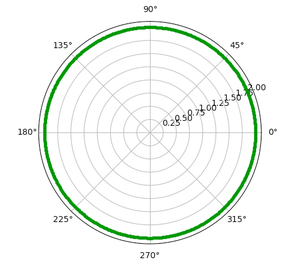2. Ellipse : An ellipse is the locus of a point moving in a plane such that the sum of its distances from two other points (the foci) is constant. Here, r is defined as :Where,

• a = length of semi major axis
• b = length of semi minor axis

Example :

Python3

 import numpy as npimport matplotlib.pyplot as pltimport math    # setting the axes# projection as polarplt.axes(projection = 'polar')  # setting the values of# semi-major and# semi-minor axesa = 4b = 3  # creating an array# containing the radian valuesrads = np.arange(0, (2 * np.pi), 0.01)  # plotting the ellipsefor rad in rads:    r = (a*b)/math.sqrt((a*np.sin(rad))**2 + (b*np.cos(rad))**2)    plt.polar(rad, r, 'g.')  # display the polar plotplt.show()

Output :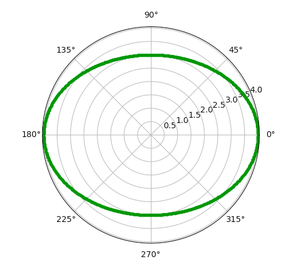3. Cardioid : A cardioid is the locus of a point on the circumference of a circle as it rolls around another identical circle. Here, r is defined as :Where, a = length of axis of cardioid

Example  :

Python3

 import numpy as npimport matplotlib.pyplot as pltimport math    # setting the axes# projection as polarplt.axes(projection = 'polar')  # setting the length of # axis of cardioida=4  # creating an array# containing the radian valuesrads = np.arange(0, (2 * np.pi), 0.01)   # plotting the cardioidfor rad in rads:    r = a + (a*np.cos(rad))     plt.polar(rad,r,'g.')   # display the polar plotplt.show()

Output :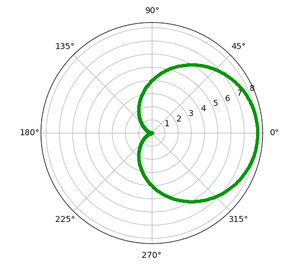4. Archimedean spiral : An Archimedean spiral is the locus of a point moving uniformly on a straight line, which itself is turning uniformly about one of its end points. Here, r is defined as :Example:

Python3

 import numpy as npimport matplotlib.pyplot as plt    # setting the axes# projection as polarplt.axes(projection = 'polar')  # creating an array# containing the radian valuesrads = np.arange(0, 2 * np.pi, 0.001)   # plotting the spiralfor rad in rads:    r = rad    plt.polar(rad, r, 'g.')      # display the polar plotplt.show()

Output :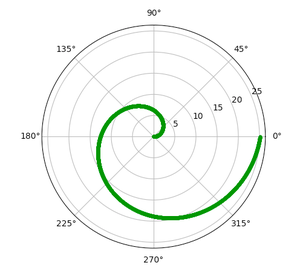5. Rhodonea : A Rhodonea or Rose curve is a rose-shaped sinusoid plotted in polar coordinates. Here, r is defined as :Where,

• a = length of petals
• n = number of petals

Example:

Python3

 import numpy as npimport matplotlib.pyplot as plt    # setting the axes# projection as polarplt.axes(projection='polar')  # setting the length# and number of petalsa = 1n = 6  # creating an array# containing the radian valuesrads = np.arange(0, 2 * np.pi, 0.001)   # plotting the rosefor rad in rads:    r = a * np.cos(n*rad)    plt.polar(rad, r, 'g.')   # display the polar plotplt.show()

Output :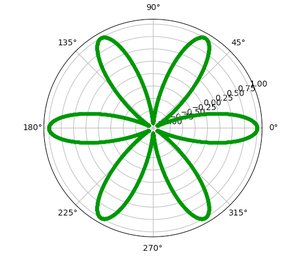Attention geek! Strengthen your foundations with the Python Programming Foundation Course and learn the basics.

To begin with, your interview preparations Enhance your Data Structures concepts with the Python DS Course. And to begin with your Machine Learning Journey, join the Machine Learning - Basic Level Course

My Personal Notes arrow_drop_up